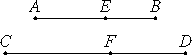# Proposition 66

A straight line commensurable in length with a binomial straight line is itself also binomial and the same in order.

Let AB be binomial, and let CD be commensurable in length with AB.

I say that CD is binomial and the same in order with AB.X.36

Since AB is binomial, divide it into its terms at E, and let AE be the greater term, therefore AE and EB are rational straight lines commensurable in square only.

Let it be contrived that AB is to CD as AE is to CF. Then the remainder EB is to the remainder FD as AB is to CD.

X.11

But AB is commensurable in length with CD, therefore AE is also commensurable with CF, and EB with FD.

And AE and EB are rational, therefore CF and FD are also rational.

And AE is to CF as EB is to FD. Therefore, alternately, AE is to EB as CF is to FD.

X.11

But AE and EB are commensurable in square only, therefore CF and FD are also commensurable in square only.

X.36

And they are rational, therefore CD is binomial.

I say next that it is the same in order with AB.

For the square on AE is greater than the square on EB either by the square on a straight line commensurable with AE or by the square on a straight line incommensurable with it.

X.14

If then the square on AE is greater than the square on EB by the square on a straight line commensurable with AE, then the square on CF is also greater than the square on FD by the square on a straight line commensurable with CF.

And, if AE is commensurable with the rational straight line set out, then CF is also commensurable with it, and for this reason each of the straight lines AB and CD is a first binomial, that is, the same in order.

But, if EB is commensurable with the rational straight line set out, then FD is also commensurable with it, and for this reason again CD is the same in order with AB, for each of them is a second binomial.

But, if neither of the straight lines AE nor EB is commensurable with the rational straight line set out, then neither of the straight lines CF nor FD is commensurable with it, and each of the straight lines AB and CD is a third binomial.

X.14

But, if the square on AE is greater than the square on EB by the square on a straight line incommensurable with AE, then the square on CF is also greater than the square on FD by the square on a straight line incommensurable with CF.

X.Def.II.4

And, if AE is commensurable with the rational straight line set out, then CF is also commensurable with it, and each of the straight lines AB and CD is a fourth binomial.

X.Def.II.5

But, if EB is so commensurable, then FD is also, and each of the straight lines AB and CD is a fifth binomial.

X.Def.II.6

But, if neither of the straight lines AE or EB is so commensurable, then neither of the straight lines CF or FD is commensurable with the rational straight line set out, and each of the straight lines AB and CD is a sixth binomial.

Hence a straight line commensurable in length with a binomial straight line is binomial and the same in order.

Therefore, a straight line commensurable in length with a binomial straight line is itself also binomial and the same in order.

Q.E.D.

## Guide

This proposition is not used in the rest of the Elements